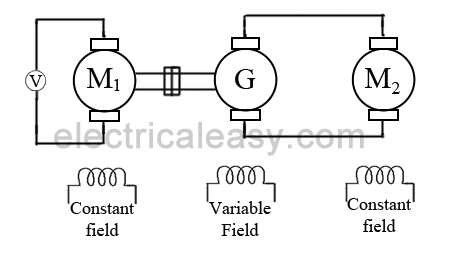# Speed control methods of DC motor

### Speed of a DC motor

Back emf Eb of a DC motor is nothing but the induced emf in armature conductors due to rotation of the armature in magnetic field. Thus, the magnitude of Eb can be given by EMF equation of a DC generator.
Eb = PØNZ/60A
(where, P = no. of poles, Ø = flux/pole, N = speed in rpm, Z = no. of armature conductors, A = parallel paths)

Eb can also be given as,
Eb = V- IaRa

thus, from the above equations
N = Eb 60A/PØZ

but, for a DC motor A, P and Z are constants

Therefore, N K Eb/Ø          (where, K=constant)

This shows the speed of a dc motor is directly proportional to the back emf and inversely proportional to the flux per pole.

## Speed control methods of DC motor

### Speed control of Shunt motor

#### 1. Flux control method

It is already explained above that the speed of a dc motor is inversely proportional to the flux per pole. Thus by decreasing the flux, speed can be increased and vice versa.
To control the flux, a rheostat is added in series with the field winding, as shown in the circuit diagram. Adding more resistance in series with the field winding will increase the speed as it decreases the flux. In shunt motors, as field current is relatively very small, Ish2R loss is small. Therefore, this method is quite efficient. Though speed can be increased above the rated value by reducing flux with this method, it puts a limit to maximum speed as weakening of field flux beyond a limit will adversely affect the commutation.

#### 2. Armature control method

Speed of a dc motor is directly proportional to the back emf Eb and Eb = V - IaRa. That means, when supply voltage V and the armature resistance Ra are kept constant, then the speed is directly proportional to armature current Ia. Thus, if we add resistance in series with the armature, Ia decreases and, hence, the speed also decreases. Greater the resistance in series with the armature, greater the decrease in speed.

#### 3. Voltage Control Method

a) Multiple voltage control:
In this method, the shunt field is connected to a fixed exciting voltage and armature is supplied with different voltages. Voltage across armature is changed with the help of suitable switchgear. The speed is approximately proportional to the voltage across the armature.

b) Ward-Leonard System:This system is used where very sensitive speed control of motor is required (e.g electric excavators, elevators etc.). The arrangement of this system is as shown in the figure at right.
M2 is the motor to which speed control is required.
M1 may be any AC motor or DC motor with constant speed.
G is a generator directly coupled to M1.
In this method, the output from generator G is fed to the armature of the motor M2 whose speed is to be controlled. The output voltage of generator G can be varied from zero to its maximum value by means of its field regulator and, hence, the armature voltage of the motor M2 is varied very smoothly. Hence, very smooth speed control of the dc motor can be obtained by this method.

### Speed control of series motor

#### 1. Flux control method• Field diverter: A variable resistance is connected parallel to the series field as shown in fig (a). This variable resistor is called as a diverter, as the desired amount of current can be diverted through this resistor and, hence, current through field coil can be decreased. Thus, flux can be decreased to the desired amount and speed can be increased.
• Armature diverter: Diverter is connected across the armature as shown in fig (b).
For a given constant load torque, if armature current is reduced then the flux must increase, as Ta ∝ ØIa
This will result in an increase in current taken from the supply and hence flux Ø will increase and subsequently speed of the motor will decrease.
• Tapped field control: As shown in fig (c) field coil is tapped dividing number of turns. Thus we can select different value of Ø by selecting different number of turns.
• Paralleling field coils: In this method, several speeds can be obtained by regrouping coils as shown in fig (d).

#### 2. Variable resistance in series with armature

By introducing resistance in series with the armature, voltage across the armature can be reduced. And, hence, speed reduces in proportion with it.

#### 3. Series-parallel control

This system is widely used in electric traction, where two or more mechanically coupled series motors are employed. For low speeds, the motors are connected in series, and for higher speeds, the motors are connected in parallel.
When in series, the motors have the same current passing through them, although voltage across each motor is divided. When in parallel, the voltage across each motor is same although the current gets divided.